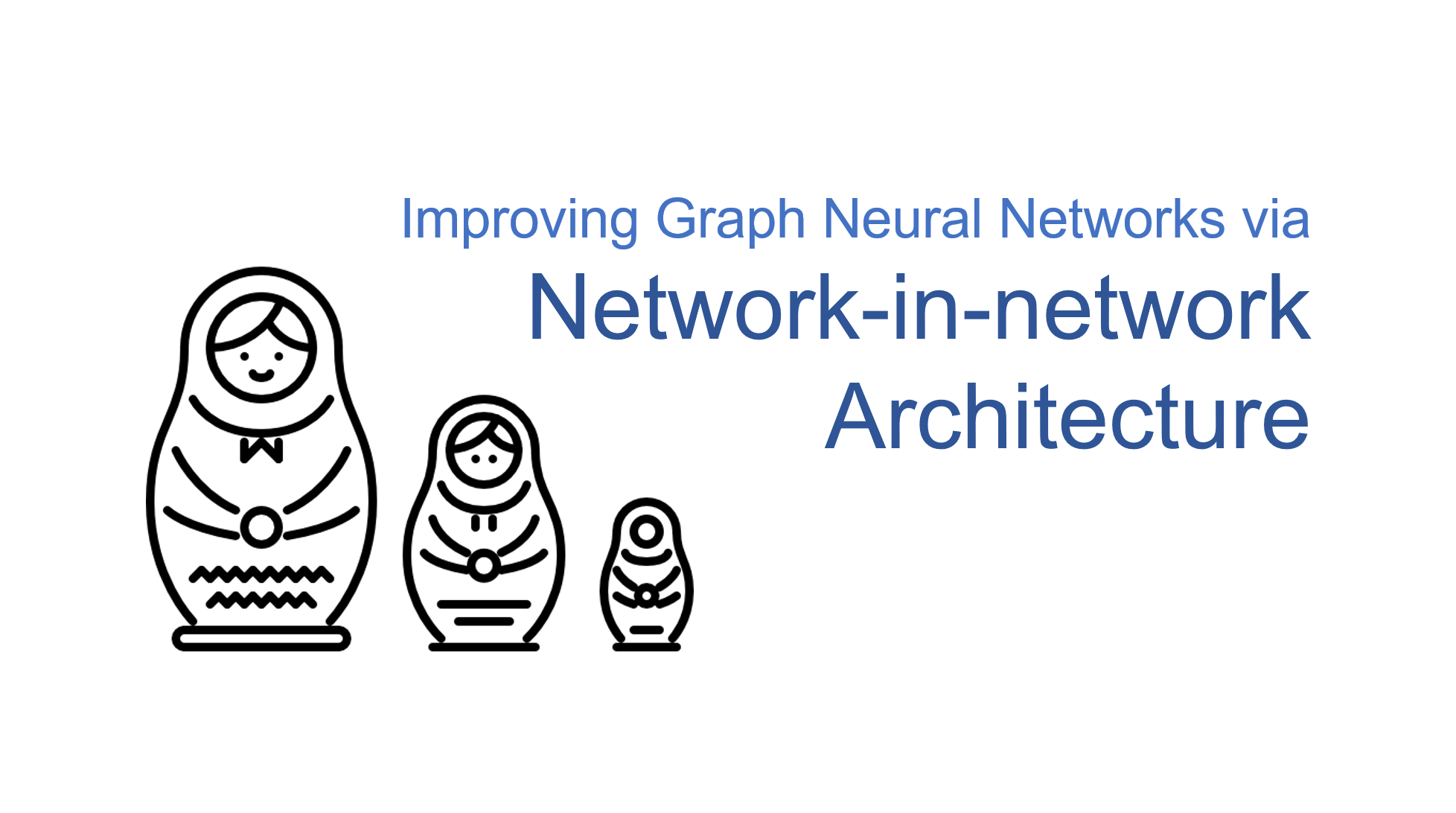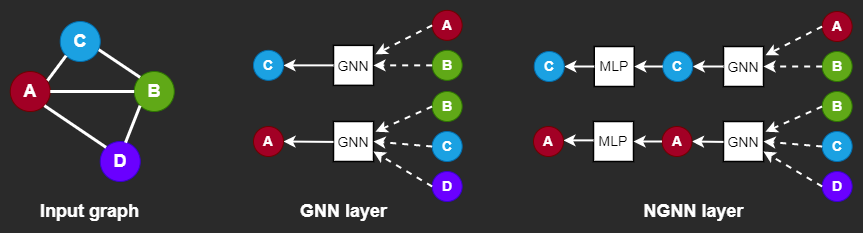## Blog Details

Home / Blog Details#### Improving Graph Neural Networks via Network-in-network Architecture

As Graph Neural Networks (GNNs) has become increasingly popular, there is a wide interest of designing deeper GNN architecture. However, deep GNNs suffer from the oversmoothing issue where the learnt node representations quickly become indistinguishable with more layers. This blog features a simple yet effective technique to build a deep GNN without the concern of oversmoothing. The new architecture, Network in Graph Neural Networks (NGNN) inspired by the network-in-network architecture for computer vision, has shown superior performance on multiple Open Graph Benchmark (OGB) leaderboards.

## Introducing NGNN

At a high-level, a graph neural network (MPGNN) layer can be written as a non-linear function:

with $h^{(0)}=X$ being the input node features, $\mathcal{G}$ being the input graph, $h^L$ being the node embeddings in the last layer used by downstream tasks, $L$ being the number of GNN layers. Additionally, the function $f_w\left(\mathcal{G}, h^l\right)$ is determined by learnable parameters $w$ and $\sigma(\cdot)$ is a non-linear activation function.

Instead of adding many more GNN layers, NGNN deepens a GNN model by inserting nonlinear feedforward neural network layer(s) within each GNN layer.In essence, NGNN is just a nonlinear transformation of the original embeddings of the nodes in the $l$-th layer. Despite its simplicity, the NGNN technique is quite powerful (we will come to that in a moment). Additionally, it does not have large memory overhead and can work with various training methods such as neighbor sampling or subgraph sampling.

The intuition behind is straightforward. As the number of GNN layers and the number of training iterations increases, the representations of nodes within the same connected component will tend to converge to the same value. NGNN uses a simple MLP after certain GNN layers to tackle the so-called oversmoothing issue.

## Implementing NGNN in Deep Graph Library (DGL)

For better gaining insights into this trick, let us use DGL to implement a simple NGNN, using the GCN layer as the backbone.

With DGL’s builtin GCN layer dgl.nn.GraphConv, we can easily implement a minimal NGNN_GCN layer, which just applies an $\rm{ReLU}$ activation and a linear transformation after a GCN layer.

from dgl.nn import GraphConv

class NGNN_GCNConv(torch.nn.Module):
def __init__(self, input_channels, hidden_channels, output_channels):
super(NGNN_GCNConv, self).__init__()
self.conv = GraphConv(input_channels, hidden_channels)
self.fc = Linear(hidden_channels, output_channels)

def forward(self, g, x, edge_weight=None):
x = self.conv(g, x, edge_weight)
x = F.relu(x)
x = self.fc(x)
return x


Afterwards, you can simply stack the dgl.nn.GraphConv layer and the NGNN_GCN layer to form a multi-layer NGNN_GCN network.

class NGNN_GCN(nn.Module):
def __init__(self, input_channels, hidden_channels, output_channels):
super(Model, self).__init__()
self.conv1 = NGNN_GCNConv(input_channels, hidden_channels, hidden_channels)
self.conv2 = GraphConv(hidden_channels, output_channels)

def forward(self, g, input_channels):
h = self.conv1(g, input_channels)
h = F.relu(h)
h = self.conv2(g, h)
return h


You can replace dgl.nn.GraphConv with any other graph convolution layers in the NGNN architecture. DGL provides implementation of many popular convolutional layers and utility modules. You can easily invoke them with one line of code and build your own NGNN modules.

## Model Performance

NGNN can be used for many downstream tasks, such as Node Classification/Regression, Edge Classification/Regression, Link prediction and Graph Classification. In general, NGNN achieves better results than its backbone GNN on these tasks. For instance, NGNN+SEAL achieves top-1 performance on the ogbl-ppa leaderboard with an improvement of Hit@100 by $10.91\%$ over the vanilla SEAL. The table below shows the performance improvement of NGNN over various vanilla GNN backbones.

Dataset Metric Model Performance
ogbn-proteins ROC-AUC(%) GraphSage+Cluster Sampling Vanilla 67.45 ± 1.21
+NGNN 68.12 ± 0.96
ogbn-products Accuracy(%) GraphSage Vanilla 78.27 ± 0.45
+NGNN 79.88 ± 0.34
GAT+Neighbor Sampling Vanilla 79.23 ± 0.16
+NGNN 79.67 ± 0.09
ogbl-collab hit@50(%) GCN Vanilla 49.52 ± 0.70
+NGNN 53.48 ± 0.40
GraphSage Vanilla 51.66 ± 0.35
+NGNN 53.59 ± 0.56
ogbl-ppa hit@100(%) SEAL-DGCNN Vanilla 48.80 ± 3.16
+NGNN 59.71 ± 2.45
GCN Vanilla 18.67 ± 1.32
+NGNN 36.83 ± 0.99